# Multiplying Tens, Hundreds and Thousands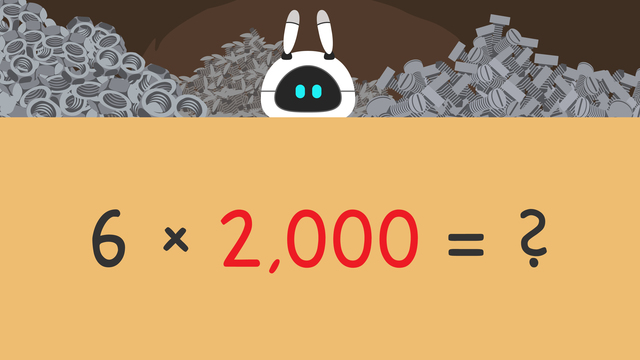Rate this video

Ø 5.0 / 2 ratings

The authorTeam Digital

## DescriptionMultiplying Tens, Hundreds and Thousands

### Contents

• How do we Multiply by 10, 100, and 1,000?
• ### How do we Multiply by 10, 100, and 1,000?

Mr. Squeaks is building a surprise for Imani and is trying to calculate how many nails, bolts, and nuts he has. In order to do that, he will practice multiplying by 10, multiplying by 100, and multiplying by 1,000. What happens to the digits when multiplying by 10, 100, and 1,000? Below, you will see there are three easy steps you can take to find the product.

#### Steps to Multiplying by 10, 100, and 1,000

• Step one: Ignore the zeros.
• Step two: Find the product of the remaining numbers,
• Step three: Count the number of zeros and add or annex them to the product.

Now that you are familiar with the steps, let’s take a look at some examples of multiplication by 10, 100, and 1,000.

#### Multiplying by 10, 100, and 1,000 Examples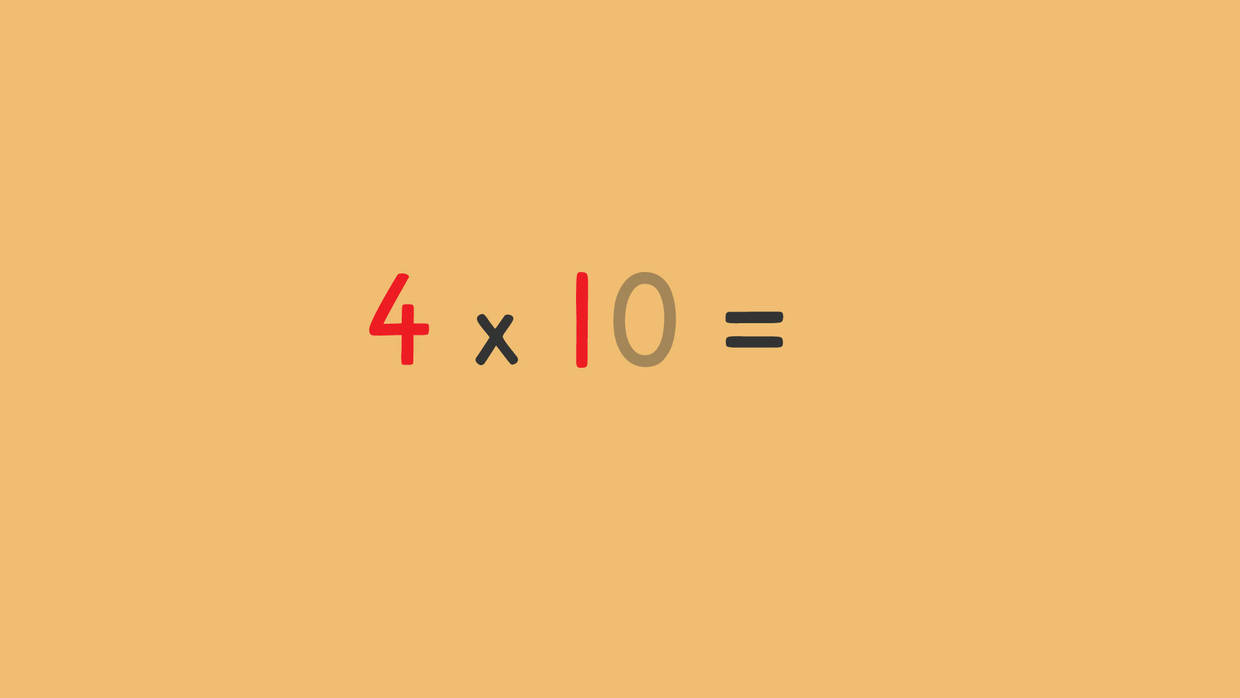What happens to the digits when you multiply by 10? Here, we are multiplying four times ten. When multiplying by 10, the first step is to ignore the zeros. The second step is to find the product of the remaining numbers four and one, which is four. The third step is to add or annex a zero to our answer. When you multiply by 10, you add just one zero to the product because there is only one zero in the problem. The product of four times ten is forty.

Let’s practice multiplying by 100 next.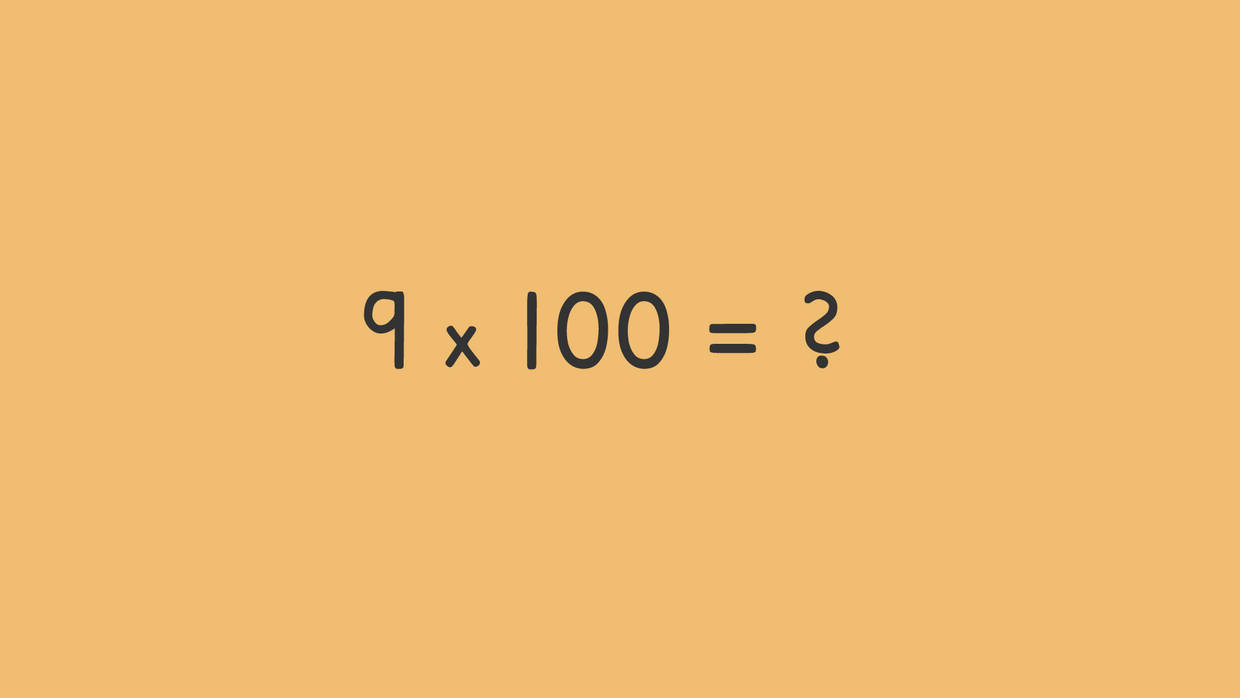What happens to the digits when you multiply by 100? To find the product of nine times one hundred, we first ignore the zeros. Next, we find the product of the remaining numbers nine and one, which is nine. Last, we add or annex the zeros to our answer. When you multiply by 100, you add two zeros to the product because there are two zeros in the problem. The product of nine times one hundred is nine hundred.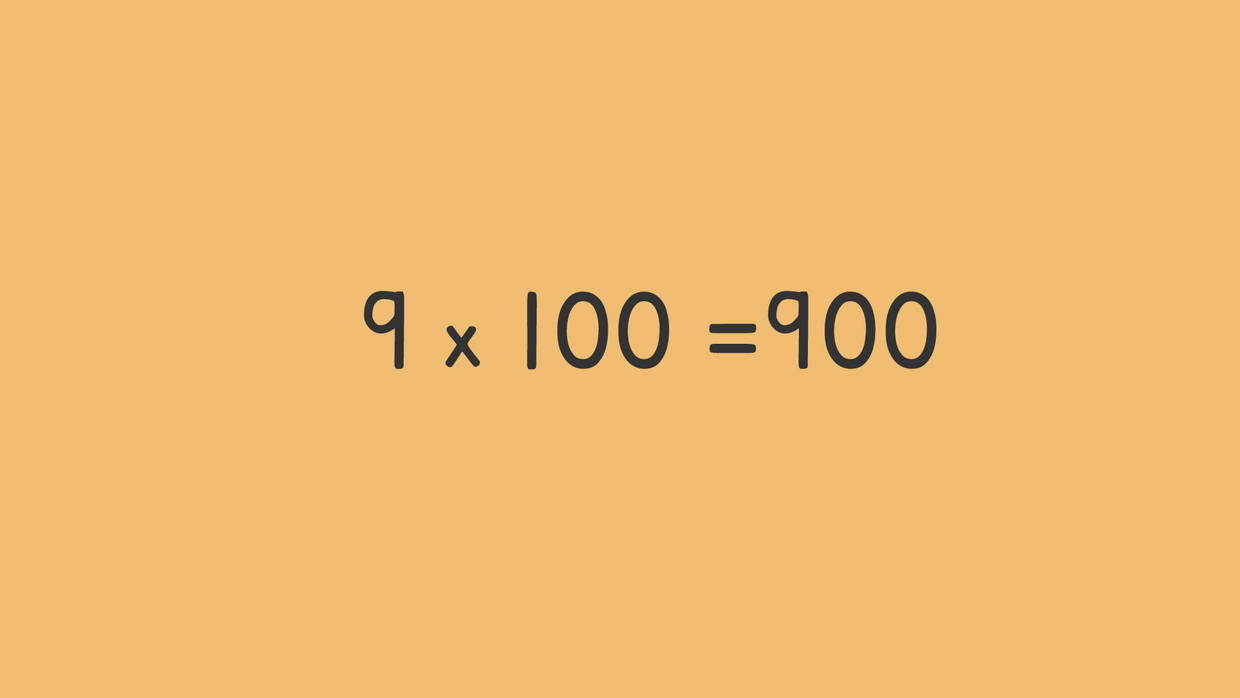Now, let’s practice multiplying by 1,000.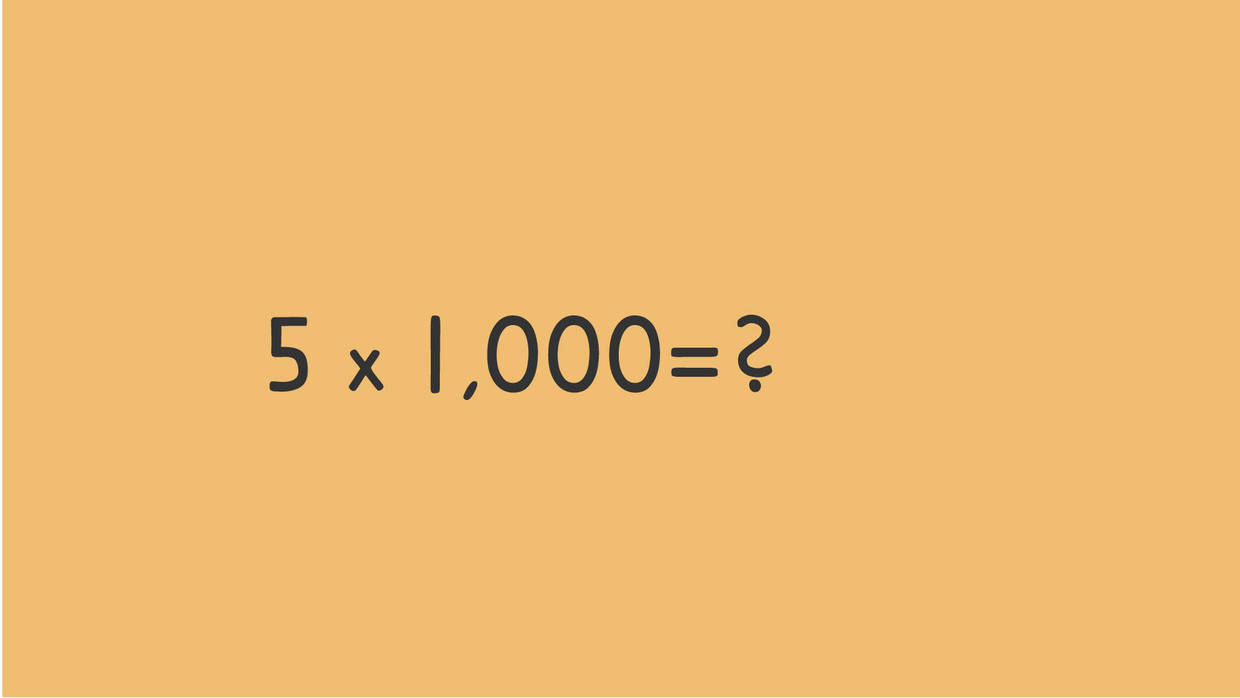What happens to the digits when you multiply by 1000? To find the product of five times one thousand, we first ignore the zeros. Next, we find the product of remaining numbers five and one, which is five. Last, we add or annex the zeros to our answer. When you multiply by 1,000, you add three zeros to the product because there are three zeros in the problem. The product of five times one thousand is five thousand.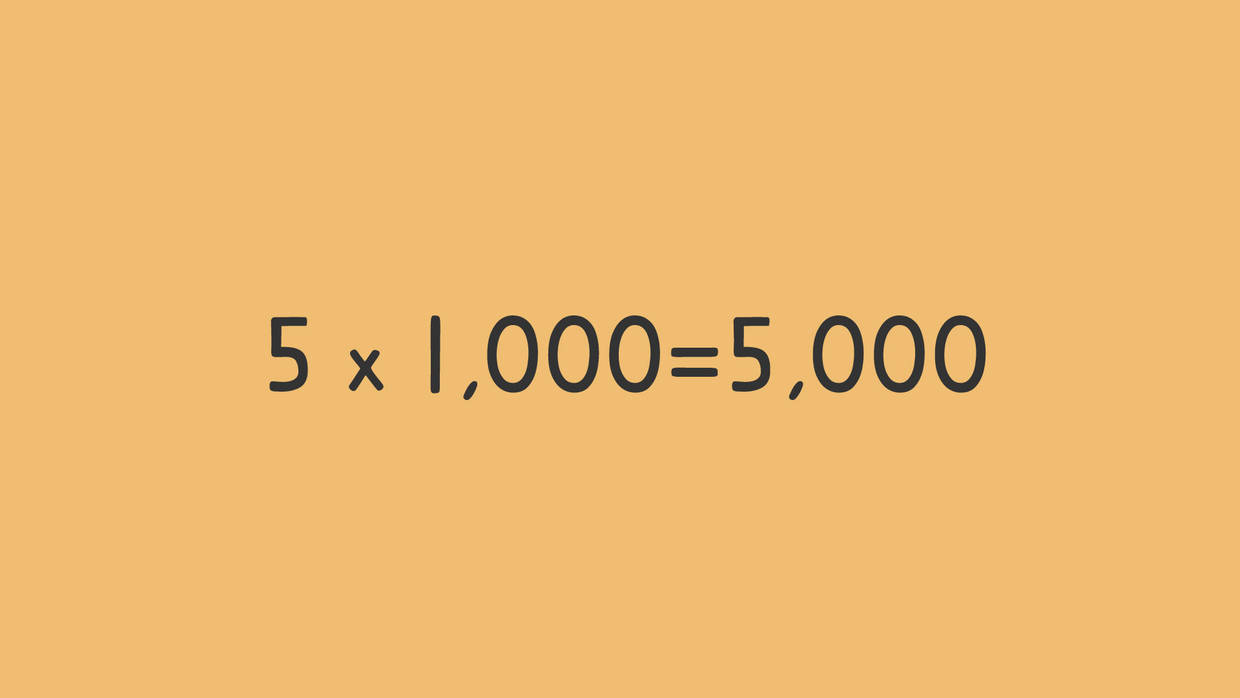#### Multiplying by 10, 100, and 1,000 Summary

Remember when multiplying by 10, 100, and 1,000 there are three easy steps you can take to find the product.

• Step one: Ignore the zeros.
• Step two: Find the product of the remaining numbers,
• Step three: Count the number of zeros and add or annex them to the product.

Have you practiced yet? On this website, you can also find multiplying by 10 100 and 1000 worksheets and exercises.

### TranscriptMultiplying Tens, Hundreds and Thousands

Mr. Squeaks is creating a surprise for Imani behind the curtain. He needs some help calculating how many nails, bolts, and nuts he has. In order to know how many of each are in the boxes we need to assist with multiplying tens, hundreds, and thousands. When you multiply a whole number by tens, hundreds, and thousands the first step is to ignore the zeros. The second step is to find the product of the remaining numbers. The third step is to count the number of zeros and add or annex them to the product. For example, in the problem four times ten, we would ignore the zero and find the product of four times one. Four times one equals four. We notice here that there is only one zero in the problem four times ten, so we add, or annex, one zero to the answer. The product of four times ten is forty. Now that you know the steps needed to multiply by tens, hundreds, and thousands. Let's see how many bolts are in this box for Mr. Squeaks. We need to find the product of nine times one hundred. What is our first step? The first step is to ignore the zeros. What is the second step? The second step is to find the product of the remaining numbers. Nine times one equals nine. Now, what do we do with the zeros that we ignored in the beginning? The third step is to count the number of zeros and annex them to the product. Since there are two zeros in the number one hundred, we annex two zeros to our answer making the product of nine times one hundred, nine hundred. Let's help Mr. Squeaks calculate how many nuts he has in this box. We need to find the product of five times one thousand. What is our first step? The first step is to ignore the zeros. What is the second step? The second step is to find the product of the remaining numbers. Five times one equals five. Now, what do we do with the zeros? The third step is to count the number of zeros and annex them to the product. Since there are three zeros in the number one thousand, we annex three zeros to our answer making the product of five times one thousand, five thousand. Let's calculate how many bolts Mr. Squeaks has in his last box. We need to find the product of six times two thousand. What is our first step? The first step is to ignore the zeros. What is the second step? The second step is to find the product of the remaining numbers. Six times two equals twelve. Now, what do we do with the zeros? The third step is to count the number of zeros and annex them to the product. Since there are three zeros in the number two thousand, we annex three zeros to our answer making the product of six times two thousand, twelve thousand. Remember, when you multiply a whole number by tens, hundreds, and thousands the first step is to ignore the zeros. The second step is to find the product of the remaining numbers. The third step is to count the number of zeros and add or annex them to the product. Phew! Mr. Squeaks has a lot of supplies! I wonder what he made for Imani...